# Registration Problem in Functional Data Analysis

##### Posted on Jan 21, 2020 (Update: Jan 29, 2020)

This post is based on the seminar, Data Acquisition, Registration and Modelling for Multi-dimensional Functional Data, given by Prof. Shi.

He illustrated with a research which is aimed at modeling patients’ recovery level after stroke via analyzing the trajectories of several bones, such as Hyoid bone, Epiglottis, head of bolus, during swallowing and other information.

The procedure of such research programme can be divided into three parts:

1. Data acquisition & preprocessing. Developed an all-in-one platform for the data acquisition, calibration, smoothing and segmentation.
2. Data registration. Introduced the GPSM method to carry out multi-dimensional functional data registration.
3. Modeling. Proposed JRC and SRC methods for simultaneous registration and classification & clustering for multi-dimensional functional data.

## Data registration using GPSM

### Landmark Registration

A point $x$ is a landmark in its neighborhood $\Omega$ if

$(\Vert D(x)\Vert > D_\mu + \lambda D_\sigma) \cap (\Vert D(x)\Vert \ge \Vert D(x')\Vert)$

for any $x’\in \Omega$.

To register query curve $w_i$ onto $w_j$ (reference curve), we need to find a warping function $h_i(t)$ such that

$w_i^*(t) = w_i[h_i(t)]\;\text{and }\; w_i^*(t_{j, landmark}) \approx w_j(t_{j,landmark})$

Warping functions usually satisfy the following conditions:

• Strict monotonicity. $h(t_1)\le h(t_2)$ for any $t_1 < t_2$
• Boundary condition. $h(0) = 0$ and $h(T)=T$
• Continuity. $h(t)$ is conditions.

### Registration: (robust) procrustes method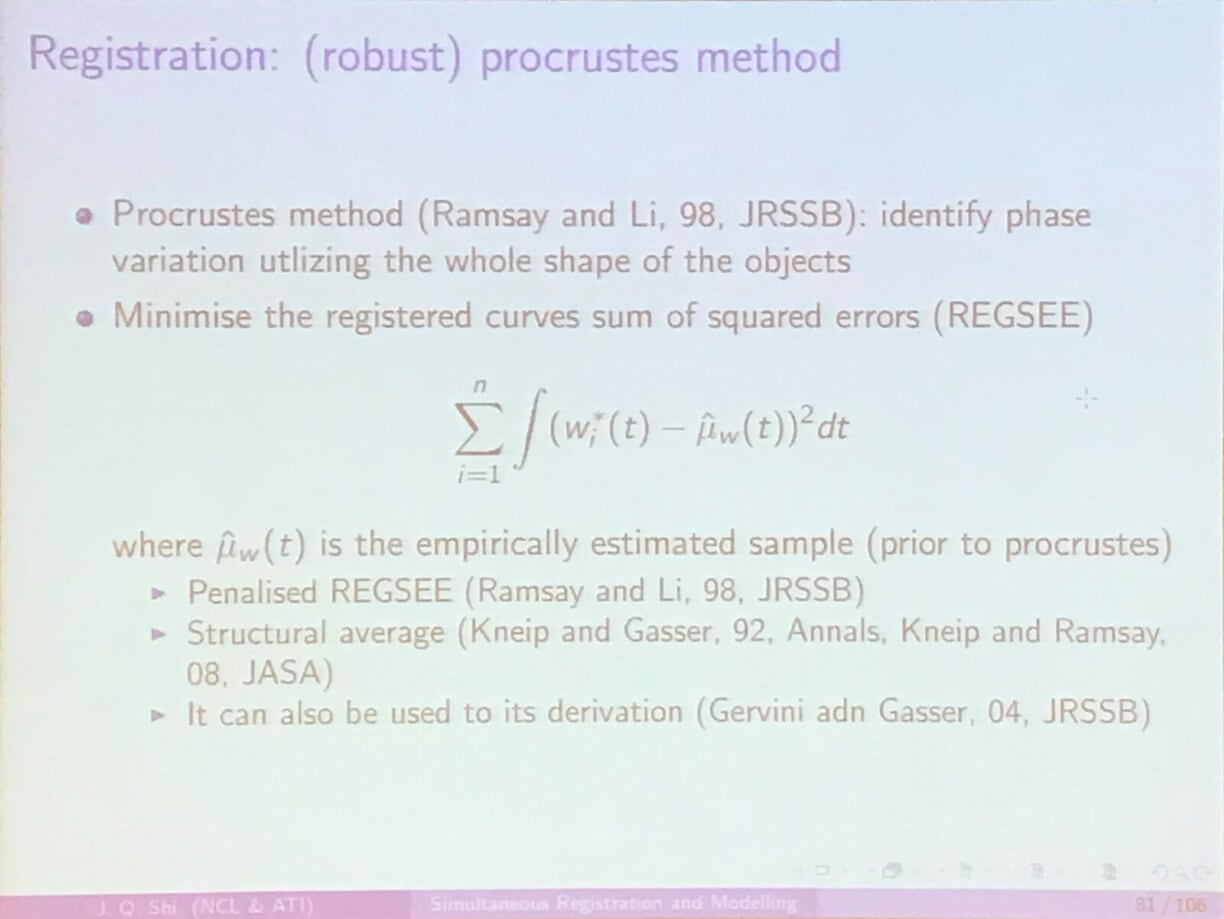what is the reference curve and the query curve

### Registration: Pairwise synchronisation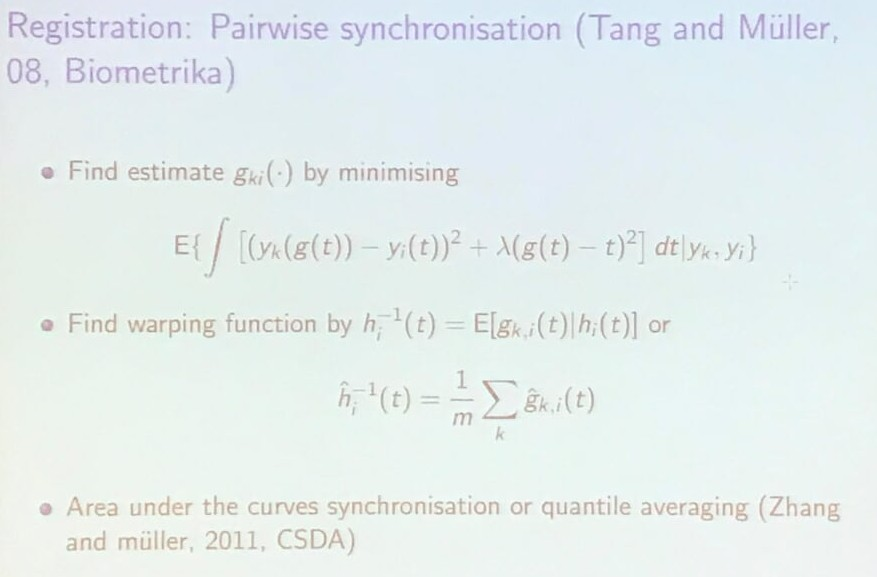### Registration: self-modeling warping function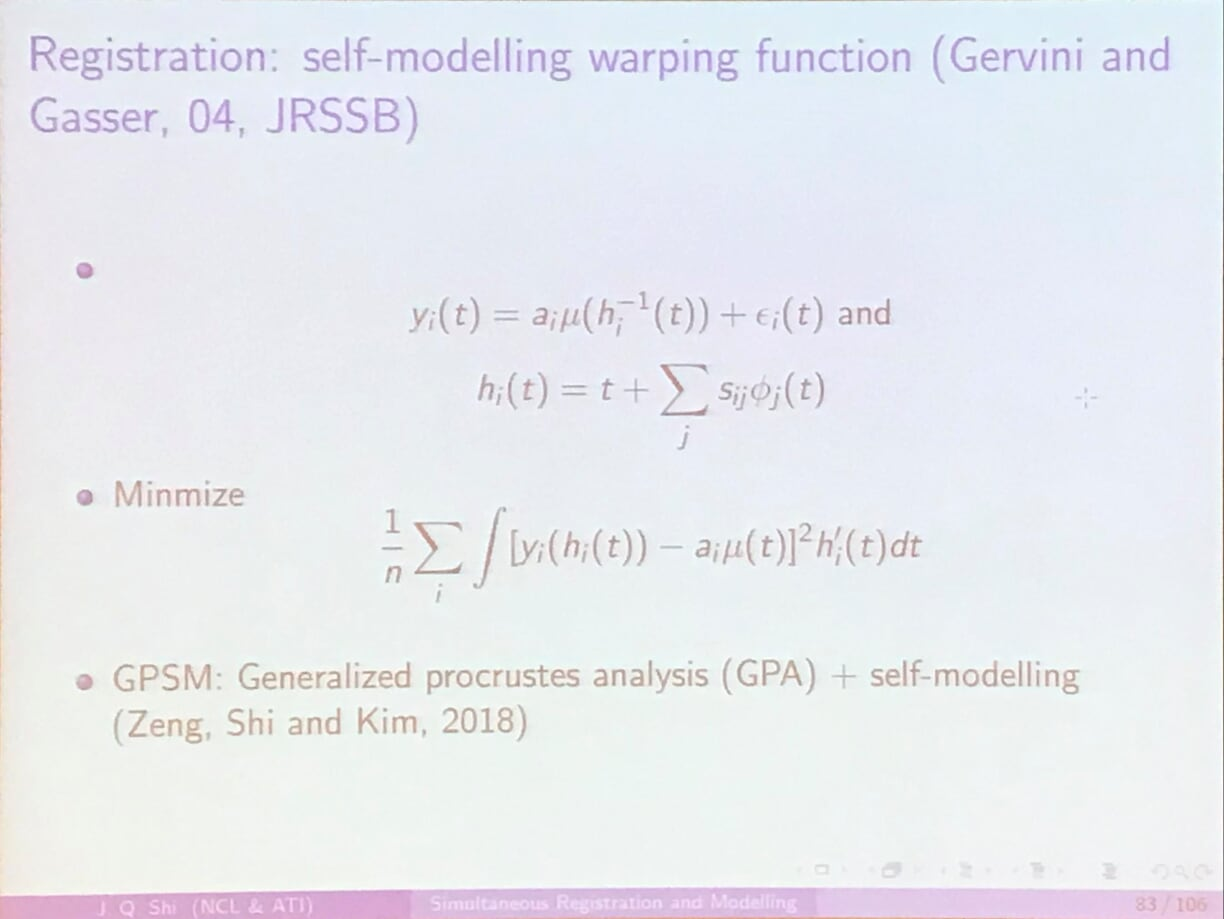### Registration: square-root velocity (SRV) function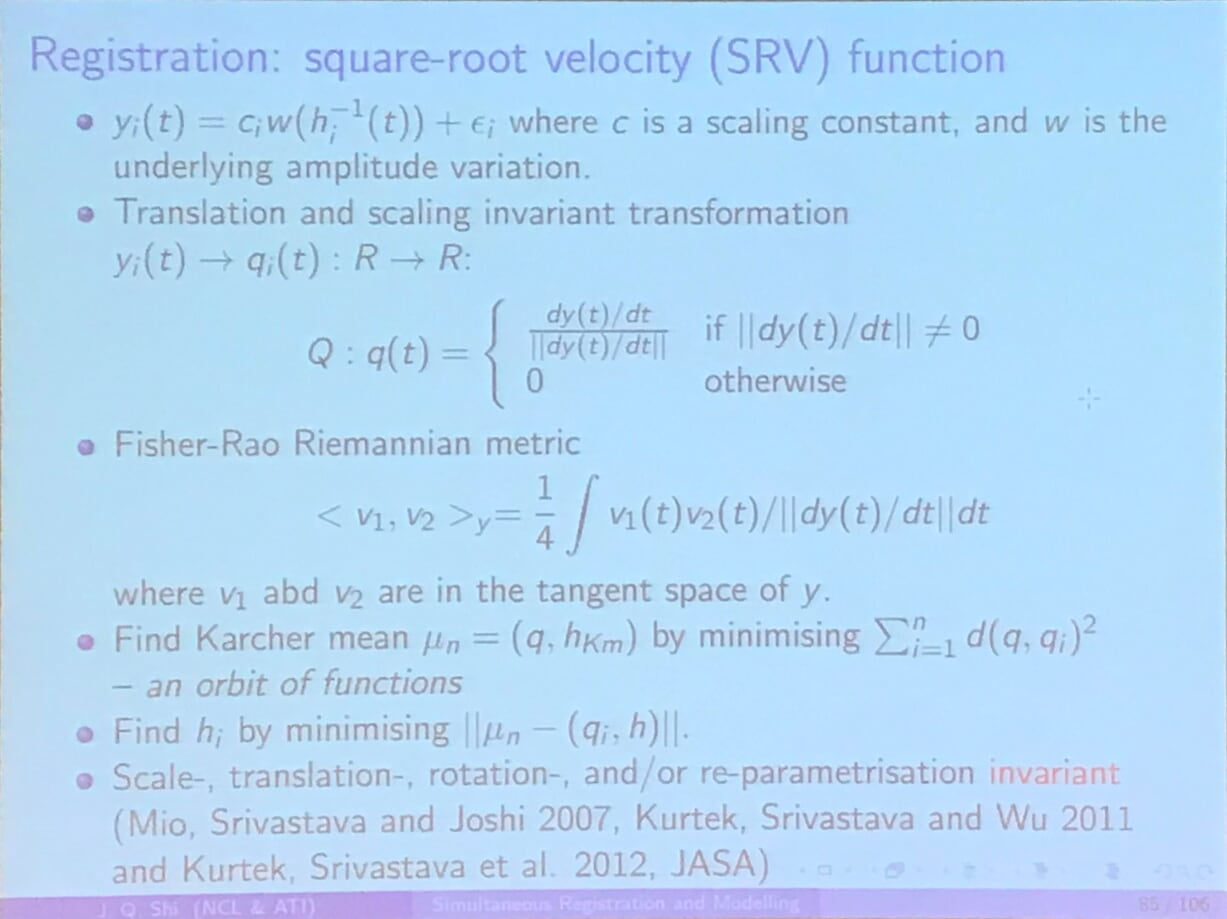## JRC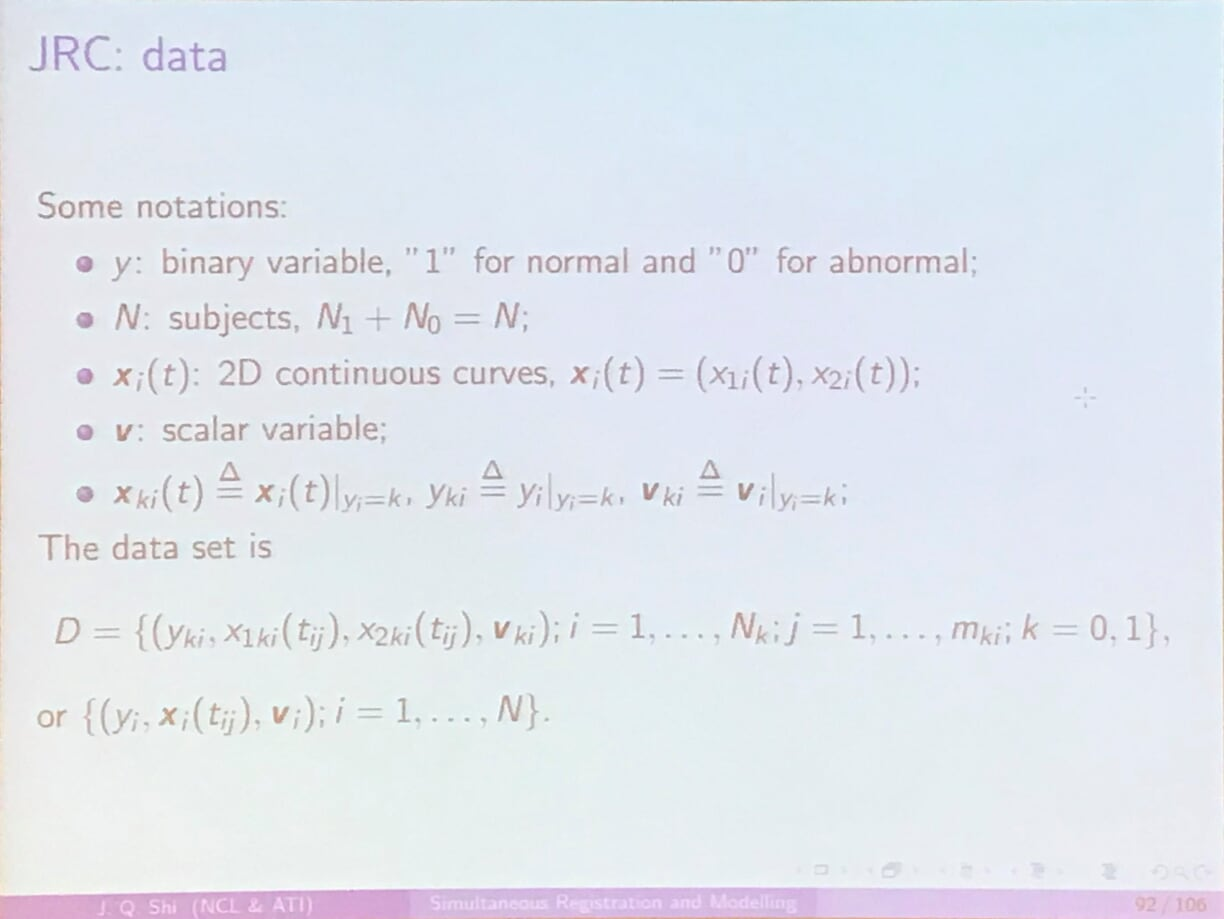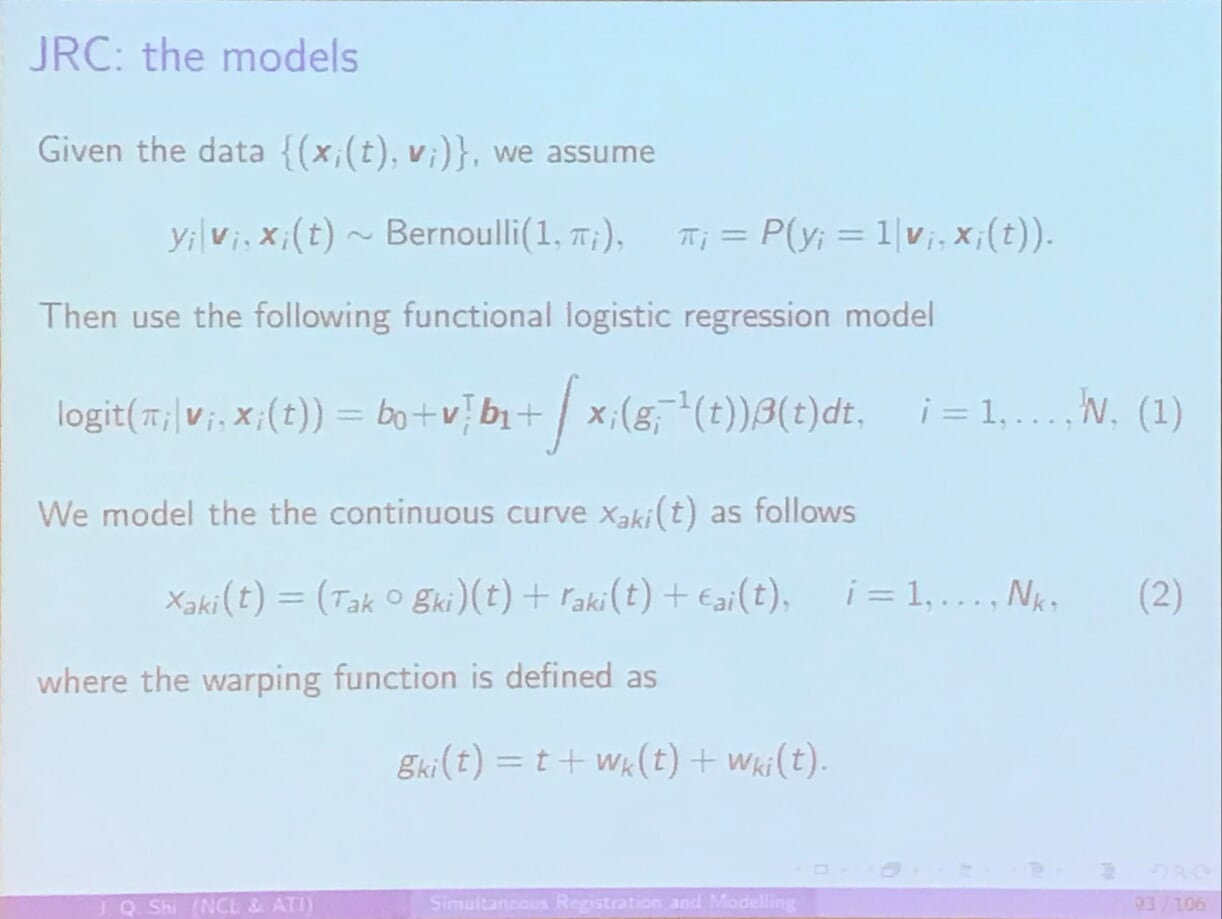## SRC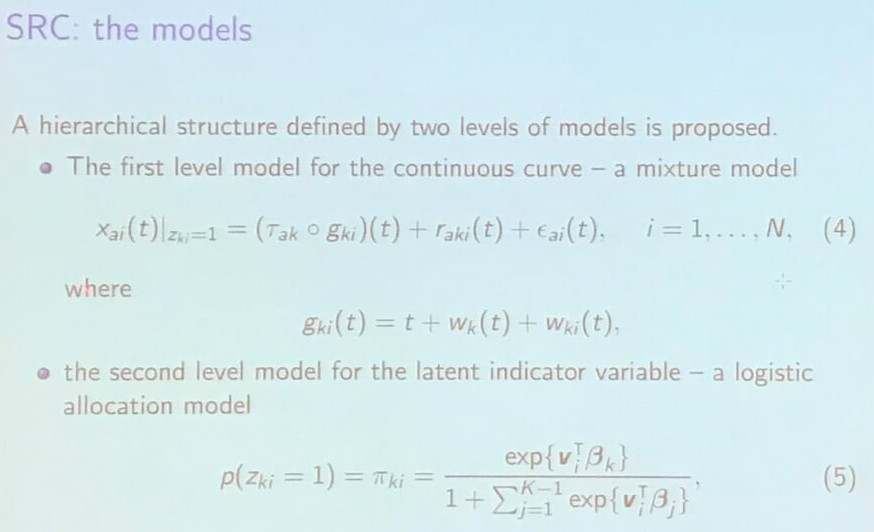How to initialize the classification? From the training? Confused about the label updating in the joint procedure?

## Conclusion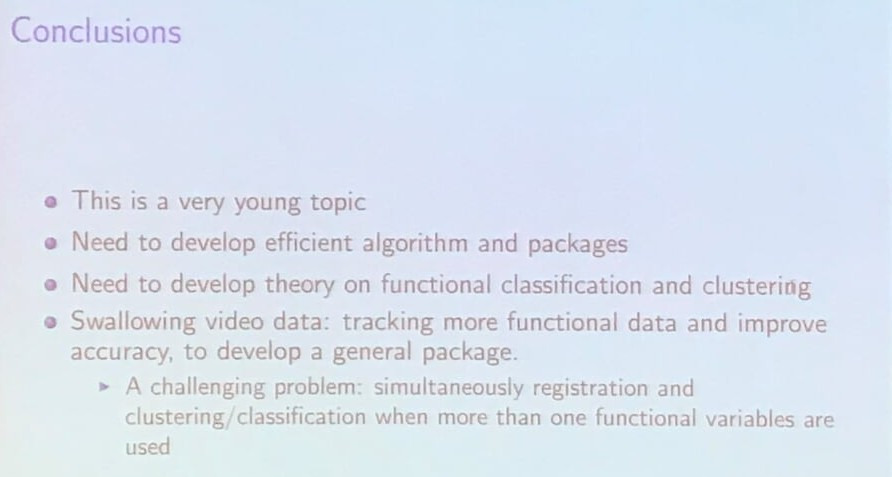Published in categories Seminar